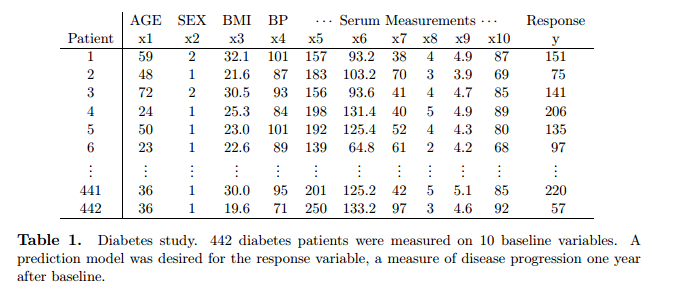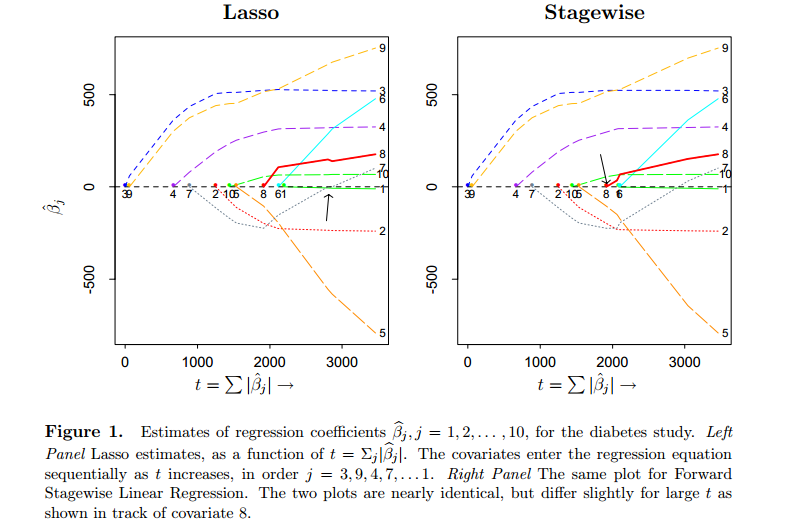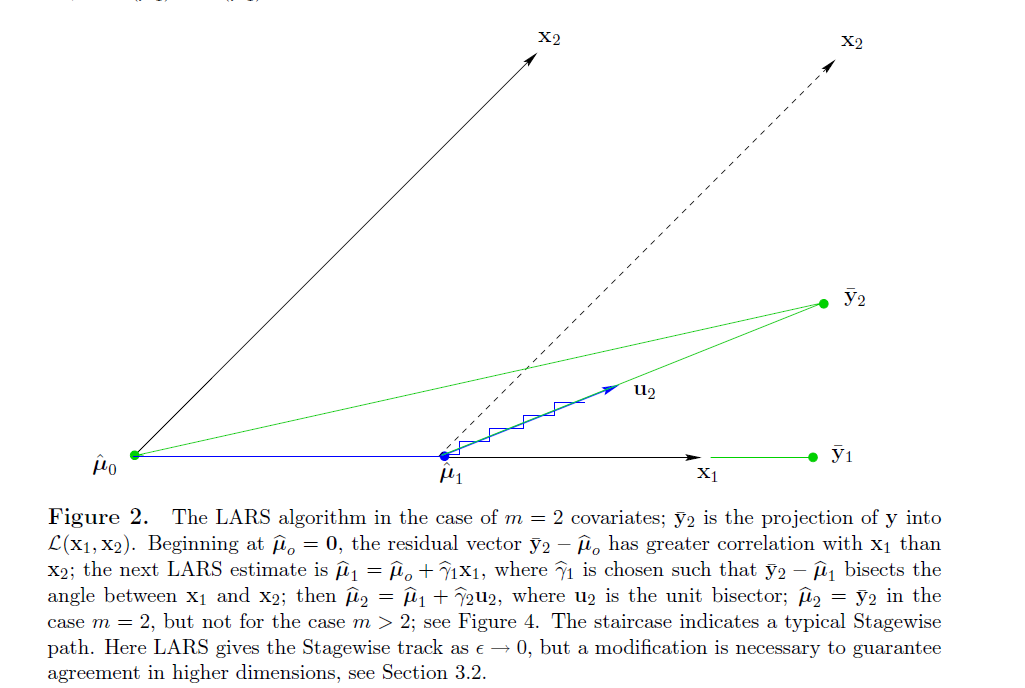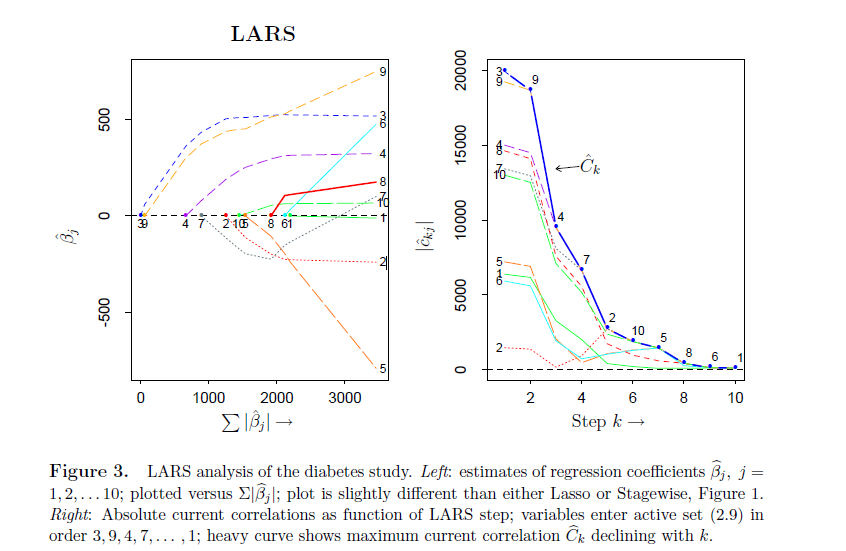# 机器学习方法：回归（三）：最小角回归Least Angle Regression（LARS），forward stagewise selection

## 问题描述X=(x1,x2,...,xn)TRn×m $X=(x_1, x_2, ...,x_n)^T \in \mathbb{R}^{n\times m}$表示数据矩阵，其中 xiRm $x_i \in \mathbb{R}^m$表示一个m维度长的数据样本； y=(y1,y2,...,yn)TRn $y=(y_1, y_2, ...,y_n)^T \in \mathbb{R}^{n}$表示数据的label，这里只考虑每个样本一类的情况。在表1的例子中， n=442m=10 $n=442，m=10$。另外，假设数据是经过一些预处理的：样本中心化并且是列单位长度的， y $y$是中心化的（减去均值），即

i=1nyi=0,i=1nxij=0,i=1nx2ij=1j=1,2,,m.

minβ^=12yμ^2,s.t.j=1m|βj^|t## forward stagewise selection

forward stagewise selection方法，下面简称为stagewise，是一个迭代算法。选择过程从 μ^=0 $\hat{\mu}=0$开始，并且不断向前走很小的step来完成回归模型（回归系数）。具体的过程如下：

c=c(μ^)=X(yμ^)

j^=argmax|c^j|andμ^μ^+ϵsign(c^j^)xj^

stagwise方法非常简单，易于实现，但是主要的问题是需要有大量的迭代步骤，因此计算量会比较大。事实上，不论是Lasso还是Stagewise方法都是Least angle regression（LARS）的变种。LARS的选择不需要经历那么多小的迭代，可以每次都在需要的方向上一步走到最远，因此计算速度很快，下面来具体描述一下LARS。

## 最小角回归Least angle regression,LARS

LARS算法也是要得到形式为μ^=Xβ^$\hat{\mu} = X\hat{\beta}$的预测值，对于m维度的数据，最多只要m步就可以把所有的维度都选上，因此在迭代次数上是非常小的。下面图2说明了LARS在2维数据下的选择过程， X=(x1x2) $X=(\text{x}_1，\text{x}_2)$c=c(μ^)=X(yμ^)=X(y¯2μ^)

μ^1=μ^0+γ^1x1

（在这里，如果是stagewise就选很小的 γ^1 $\hat{\gamma}_1$；而如果是Forward Selection，会选择一个足够大的 γ^1 $\hat{\gamma}_1$使得 μ^1=y¯1 $\hat{\mu}_1 = \bar{y}_1$,即 y $y$x1$\text{x}_1$方向上的投影。）LARS会选择上面两个情况的一个中间结果——刚好使得 y¯2μ^1 $\bar{y}_2 - \hat{\mu}_1$可以平分 x1 $\text{x}_1$ x2 $\text{x}_2$之间的夹角，因此， c1(μ^1)=c2(μ^1) $c_1(\hat{\mu}_1) = c_2(\hat{\mu}_1)$。 图2中可以看到上面的选择结果， y¯2μ^1 $\bar{y}_2 - \hat{\mu}_1$是坐落在单位向量 u2 $\text{u}_2$的方向上的。下一步LARS的更新方向是：
μ^2=μ^1+γ^2u2

m=2 $m=2$的情况下， γ^2 $\hat{\gamma}_2$是需要选择合适的大小使得 μ^2=y¯2 $\hat{\mu}_2 = \bar{y}_2$，得到线性回归的结果。如果 m>2 $m>2$的情况下，LARS会继续探索更多的方向。图2中阶梯线表示stagewise的一个迭代过程，最后也攀爬到 y¯2 $\bar{y}_2$。因此，其实LARS和stagewise的区别就在于，我们是可以计算出在一个方向上需要走多远的。

XA=(sjxj)jA

uA=XAwA,wA=AAG1A1A

XTAuA=AA1A,anduA2=1

1、首先 uA $u_\mathbf{A}$肯定可以表示成 XA $X_{\mathbf{A}}$的线性组合形式 uA=XAwA $u_\mathbf{A} = X_{\mathbf{A}}w_\mathbf{A}$,这里向量 wA $w_\mathbf{A}$还是未知的；
2、 uA $u_\mathbf{A}$平分 XA $X_{\mathbf{A}}$，也就是说

XTAuA=XTAXAwA=z1A

z21TA(XTAXA)1XTAXA(XTAXA)11A=1z=(1TA(XTAXA)11A)1/2

c^=c(μ^A)=X(yμ^A)

C^=maxj{|c^j|}andA={j:|c^j|=C^}

XA=(sjxj)jA

AA=(1TAG1A1A)1/2,GA=XTAXA

uA=XAwA,wA=AAG1A1A

a=XuA

μ^A+=μ^A+γ^uA

γ^=min+jAcC^c^jAAaj,C^+c^jAA+aj.

μ(γ)=μ^A+γuA

cj(γ)=xTj(yμ(γ))=xTj(y(μ^A+γuA))=c^jγaj

|cj(γ)|=C^γ(XTAXAwA)j=C^γAA

γ^=min+jAcC^c^jAAaj,C^+c^jAA+aj.|c^kj|=|xj(yμ^k1)|

μ^A+=μ^A+γ^uA=Xβ^A+γ^XAwA=X(β^A+γ^δA)

 dengcai， Unsupervised Feature Selection for Multi-cluster Data，KDD2010
 The Elements of Statistical Learning

05-1508-181万+
01-051177
08-07
01-271394
01-021744
11-096768
09-10726
07-01663
03-041135
08-081713
07-263328
01-052万+
08-022万+
07-195478
02-131121
06-223476
07-01293
06-05650